# Enable fully homomorphic encryption with Amazon SageMaker endpoints for secure, real-time inferencing

This is joint post co-written by Leidos and AWS. Leidos is a FORTUNE 500 science and technology solutions leader working to address some of the world’s toughest challenges in the defense, intelligence, homeland security, civil, and healthcare markets.

Leidos has partnered with AWS to develop an approach to privacy-preserving, confidential machine learning (ML) modeling where you build cloud-enabled, encrypted pipelines.

Homomorphic encryption is a new approach to encryption that allows computations and analytical functions to be run on encrypted data, without first having to decrypt it, in order to preserve privacy in cases where you have a policy that states data should never be decrypted. Fully homomorphic encryption (FHE) is the strongest notion of this type of approach, and it allows you to unlock the value of your data where zero-trust is key. The core requirement is that the data needs to be able to be represented with numbers through an encoding technique, which can be applied to numerical, textual, and image-based datasets. Data using FHE is larger in size, so testing must be done for applications that need the inference to be performed in near-real time or with size limitations. It’s also important to phrase all computations as linear equations.

In this post, we show how to activate privacy-preserving ML predictions for the most highly regulated environments. The predictions (inference) use encrypted data and the results are only decrypted by the end consumer (client side).

To demonstrate this, we show an example of customizing an Amazon SageMaker Scikit-learn, open sourced, deep learning container to enable a deployed endpoint to accept client-side encrypted inference requests. Although this example shows how to perform this for inference operations, you can extend the solution to training and other ML steps.

Endpoints are deployed with a couple clicks or lines of code using SageMaker, which simplifies the process for developers and ML experts to build and train ML and deep learning models in the cloud. Models built using SageMaker can then be deployed as real-time endpoints, which is critical for inference workloads where you have real time, steady state, low latency requirements. Applications and services can call the deployed endpoint directly or through a deployed serverless Amazon API Gateway architecture. To learn more about real-time endpoint architectural best practices, refer to Creating a machine learning-powered REST API with Amazon API Gateway mapping templates and Amazon SageMaker. The following figure shows both versions of these patterns.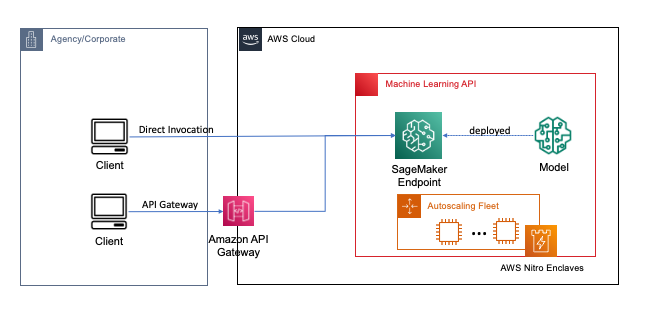In both of these patterns, encryption in transit provides confidentiality as the data flows through the services to perform the inference operation. When received by the SageMaker endpoint, the data is generally decrypted to perform the inference operation at runtime, and is inaccessible to any external code and processes. To achieve additional levels of protection, FHE enables the inference operation to generate encrypted results for which the results can be decrypted by a trusted application or client.

## More on fully homomorphic encryption

FHE enables systems to perform computations on encrypted data. The resulting computations, when decrypted, are controllably close to those produced without the encryption process. FHE can result in a small mathematical imprecision, similar to a floating point error, due to noise injected into the computation. It’s controlled by selecting appropriate FHE encryption parameters, which is a problem-specific, tuned parameter. For more information, check out the video How would you explain homomorphic encryption?

The following diagram provides an example implementation of an FHE system.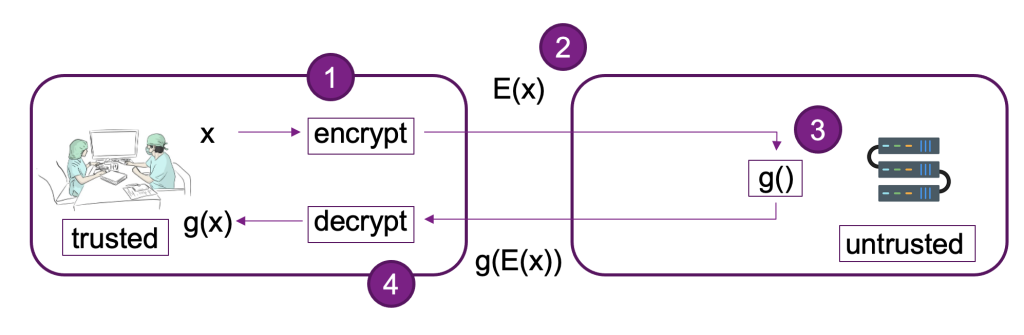In this system, you or your trusted client can do the following:

1. Encrypt the data using a public key FHE scheme. There are a couple of different acceptable schemes; in this example, we’re using the CKKS scheme. To learn more about the FHE public key encryption process we chose, refer to CKKS explained.
2. Send client-side encrypted data to a provider or server for processing.
3. Perform model inference on encrypted data; with FHE, no decryption is required.
4. Encrypted results are returned to the caller and then decrypted to reveal your result using a private key that’s only available to you or your trusted users within the client.

We’ve used the preceding architecture to set up an example using SageMaker endpoints, Pyfhel as an FHE API wrapper simplifying the integration with ML applications, and SEAL as our underlying FHE encryption toolkit.

## Solution overview

We’ve built out an example of a scalable FHE pipeline in AWS using an SKLearn logistic regression deep learning container with the Iris dataset. We perform data exploration and feature engineering using a SageMaker notebook, and then perform model training using a SageMaker training job. The resulting model is deployed to a SageMaker real-time endpoint for use by client services, as shown in the following diagram.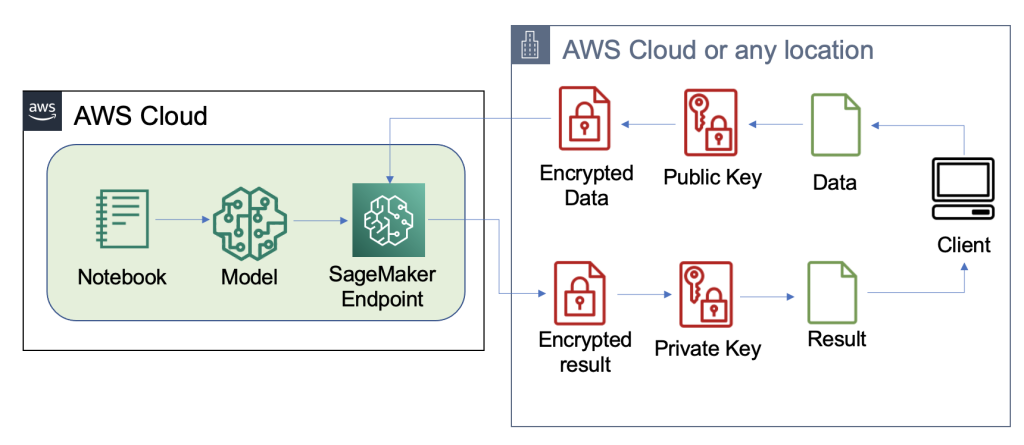In this architecture, only the client application sees unencrypted data. The data processed through the model for inferencing remains encrypted throughout its lifecycle, even at runtime within the processor in the isolated AWS Nitro Enclave. In the following sections, we walk through the code to build this pipeline.

## Prerequisites

To follow along, we assume you have launched a SageMaker notebook with an AWS Identity and Access Management (IAM) role with the AmazonSageMakerFullAccess managed policy.

## Train the model

The following diagram illustrates the model training workflow.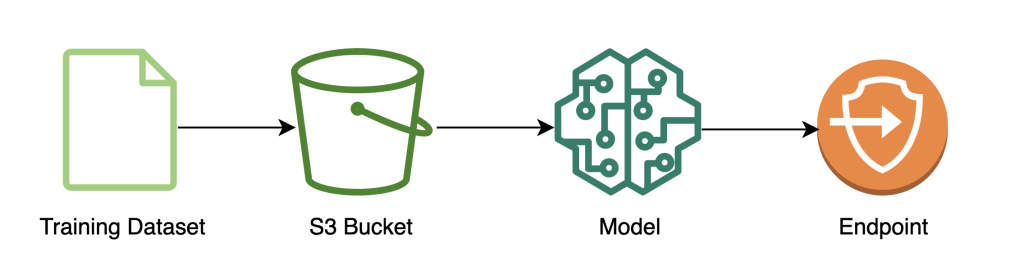The following code shows how we first prepare the data for training using SageMaker notebooks by pulling in our training dataset, performing the necessary cleaning operations, and then uploading the data to an Amazon Simple Storage Service (Amazon S3) bucket. At this stage, you may also need to do additional feature engineering of your dataset or integrate with different offline feature stores.

``````# Setup/Train Logistic Regression Estimator

# load and preprocess iris dataset
iris_df = pd.DataFrame(data=iris.data, columns=iris.feature_names)
target_df = pd.DataFrame(data=iris.target, columns=["species"])
iris_df = (iris_df - iris_df.mean(0)) / (iris_df.std(0))

# save iris dataset
iris_df = pd.concat([iris_df, target_df], axis=1)
fname = "iris.csv"
iris_df.to_csv(fname, index=False)

bucket = Session().default_bucket()
upload _path = f"training data/fhe train.csv"

In this example, we’re using script-mode on a natively supported framework within SageMaker (scikit-learn), where we instantiate our default SageMaker SKLearn estimator with a custom training script to handle the encrypted data during inference. To see more information about natively supported frameworks and script mode, refer to Use Machine Learning Frameworks, Python, and R with Amazon SageMaker.

``````# instantiate estimator
sklearn estimator = SKLearn(
role=get_execution_ role(),
entry point="fhe train.py",
source_dir="fhe_files",
instance_type="ml.c4.xlarge"
framework version="0.23-1",
)``````

Finally, we train our model on the dataset and deploy our trained model to the instance type of our choice.

``````# fit the model
sklearn estimator.fit("s3://" + bucket + "/training data")

# construct predictor from trained model
predictor = sklearn_estimator.deploy(instance_type="ml.c4.xlarge", initial_instance_count=1)``````

At this point, we’ve trained a custom SKLearn FHE model and deployed it to a SageMaker real-time inference endpoint that’s ready accept encrypted data.

## Encrypt and send client data

The following diagram illustrates the workflow of encrypting and sending client data to the model.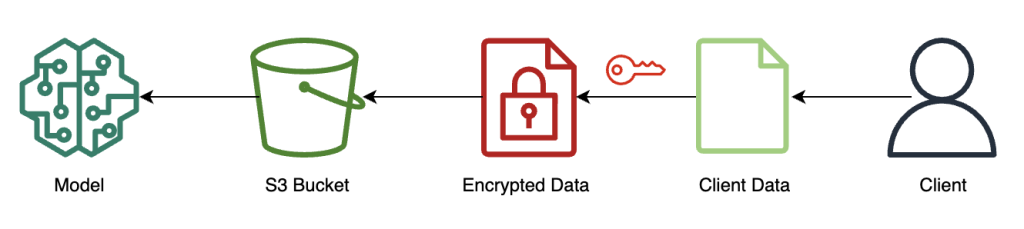In most cases, the payload of the call to the inference endpoint contains the encrypted data rather than storing it in Amazon S3 first. We do this in this example because we’ve batched a large number of records to the inference call together. In practice, this batch size will be smaller or batch transform will be used instead. Using Amazon S3 as an intermediary isn’t required for FHE.

Now that the inference endpoint has been set up, we can start sending data over. We normally use different test and training datasets, but for this example we use the same training dataset.

First, we load the Iris dataset on the client side. Next, we set up the FHE context using Pyfhel. We selected Pyfhel for this process because it’s simple to install and work with, includes popular FHE schemas, and relies upon trusted underlying open-sourced encryption implementation SEAL. In this example, we send the encrypted data, along with public keys information for this FHE scheme, to the server, which enables the endpoint to encrypt the result to send on its side with the necessary FHE parameters, but doesn’t give it the ability to decrypt the incoming data. The private key remains only with the client, which has the ability to decrypt the results.

``````# Encrypt Data Locally

# load and preprocess potentially private data
iris_df = pd.DataFrame(data=iris.data, columns=iris.feature_names)
target_df = pd.DataFrame(data=iris.target, columns=["species"])
iris_df = (iris_df - iris_df.mean(0)) / (iris_df.std(0))

# setup FHE
HE = Pyfhel()
ckks_params = {
“scheme”: "CKKS",
"n": 2 ** 14,
"scale": 2 ** 30,
"qi_sizes": [60, 30, 30, 30, 60],
}
HE.contextGen(**ckks_params)
HE.keyGen()
HE.rotateKeyGen()

# encrypt the data
iris_np = np.append(
iris_df.to_numpy(), np.ones((len(iris_df), 1)), -1 # append 1 for bias term
)
encrypted_iris = [HE.encryptFrac(row).to_bytes() for row in iris np]``````

After we encrypt our data, we put together a complete data dictionary—including the relevant keys and encrypted data—to be stored on Amazon S3. Aferwards, the model makes its predictions over the encrypted data from the client, as shown in the following code. Notice we don’t transmit the private key, so the model host isn’t able to decrypt the data. In this example, we’re passing the data through as an S3 object; alternatively, that data may be sent directly to the Sagemaker endpoint. As a real-time endpoint, the payload contains the data parameter in the body of the request, which is mentioned in the SageMaker documentation.

``````# Send data to get prediction

# store data and encryption paramters
data dict = {
"data": encrypted_iris,
"public_key": HE.to_bytes_public_key(),
"relin_key": HE.to_bytes_relin key(),
"rotate_key": HE.to_bytes_rotate_key(),
"context": HE.to_bytes_context(),
}

# upload data and encryption parameters to s3
pickle.dump(data_dict, open("request.pkl”, "wb"))

# get predictions from our instance
response = predictor.predict({ "bucket": bucket, "uri": "request.pkl"})

The following screenshot shows the central prediction within `fhe_train.py` (the appendix shows the entire training script).

``````predictions = []
for data in encrypted_data:
encrypted_prediction = [
HE.scalar_prod_plain(data, encoded_coef, in_new_ctxt=True).to_bytes()
for encoded_coef in encoded_coefs
]
predictions.append(encrypted_prediction)
``````

We’re computing the results of our encrypted logistic regression. This code computes an encrypted scalar product for each possible class and returns the results to the client. The results are the predicted logits for each class across all examples.

## Client returns decrypted results

The following diagram illustrates the workflow of the client retrieving their encrypted result and decrypting it (with the private key that only they have access to) to reveal the inference result.In this example, results are stored on Amazon S3, but generally this would be returned through the payload of the real-time endpoint. Using Amazon S3 as an intermediary isn’t required for FHE.

The inference result will be controllably close to the results as if they had computed it themselves, without using FHE.

``````# Decrypt results locally

# extract predictions from class probabilities
cl_preds = []
for prediction in predictions:
logits = [PyCtxt(bytestring=p, scheme="CKKS", pyfhel=HE) for p in prediction]
cl = np.argmax([HE.decryptFrac(logit) for logit in logits])
c1_preds.append(cl)

# compute accuracy
np.mean(cl_preds == target_df.to_numpy().squeeze()) * 100

# clean up
predictor.delete_endpoint()``````

## Clean up

We end this process by deleting the endpoint we created, to make sure there isn’t any unused compute after this process.

## Results and considerations

One of the common drawbacks of using FHE on top of models is that it adds computational overhead, which—in practice—makes the resulting model too slow for interactive use cases. But, in cases where the data is highly sensitive, it might be worthwhile to accept this latency trade-off. However, for our simple logistic regression, we are able to process 140 input data samples within 60 seconds and see linear performance. The following chart includes the total end-to-end time, including the time performed by the client to encrypt the input and decypt the results. It also uses Amazon S3, which adds latency and isn’t required for these cases.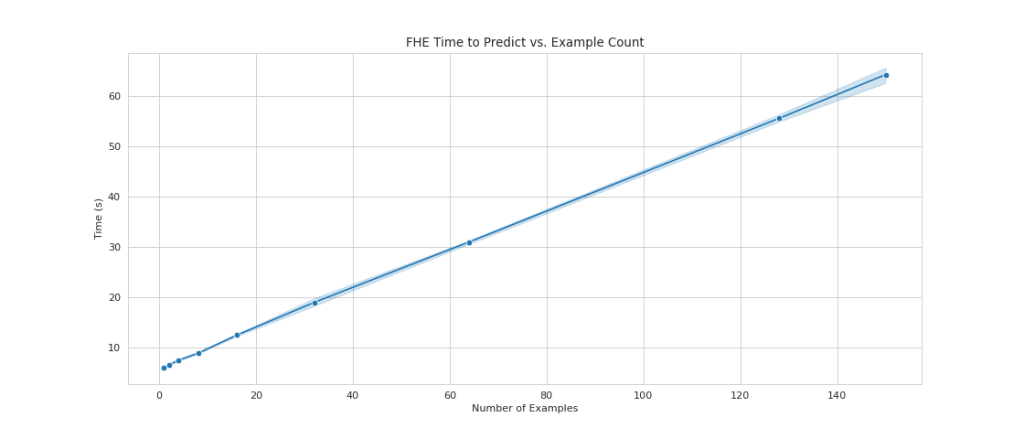We see linear scaling as we increase the number of examples from 1 to 150. This is expected because each example is encrypted independently from each other, so we expect a linear increase in computation, with a fixed setup cost.

This also means that you can scale your inference fleet horizontally for greater request throughput behind your SageMaker endpoint. You can use Amazon SageMaker Inference Recommender to cost optimize your fleet depending on your business needs.

## Conclusion

And there you have it: fully homomorphic encryption ML for a SKLearn logistic regression model that you can set up with a few lines of code. With some customization, you can implement this same encryption process for different model types and frameworks, independent of the training data.

If you’d like to learn more about building an ML solution that uses homomorphic encryption, reach out to your AWS account team or partner, Leidos, to learn more. You can also refer to the following resources for more examples:

The content and opinions in this post contains those from third-party authors and AWS is not responsible for the content or accuracy of this post.

## Appendix

The full training script is as follows:

``````import argparse
import os
import pickle
from io import BytesIO

import boto3
import joblib
import numpy as np
import pandas as pd
from Pyfhel import PyCtxt, Pyfhel
from sklearn.linear_model import LogisticRegression

def model_fn(model_dir):
return clf

def input_fn(request_body, request_content_type):
)

def predict_fn(input_data, model):
HE = Pyfhel()

data = input_data["data"]
HE.from_bytes_context(input_data["context"])
HE.from_bytes_public_key(input_data["public_key"])
HE.from_bytes_relin_key(input_data["relin_key"])
HE.from_bytes_rotate_key(input_data["rotate_key"])

encrypted_data = [PyCtxt(bytestring=row, scheme="CKKS", pyfhel=HE) for row in data]

coefs = [
np.append(coef, intercept).astype("float64")
for coef, intercept in zip(model.coef_, model.intercept_)
]
encoded_coefs = [HE.encodeFrac(coef) for coef in coefs]

predictions = []
for data in encrypted_data:
encrypted_prediction = [
HE.scalar_prod_plain(data, encoded_coef, in_new_ctxt=True).to_bytes()
for encoded_coef in encoded_coefs
]
predictions.append(encrypted_prediction)

encoded_output = pickle.dumps(predictions)
output = np.frombuffer(encoded_output, dtype="byte")

return output

if __name__ == "__main__":

parser = argparse.ArgumentParser()

# Data and model directories
"--train", type=str, default=os.environ.get("SM_CHANNEL_TRAINING")
)

args, _ = parser.parse_known_args()
train_df = pd.read_csv(args.train + "/" + "fhe_train.csv")

model = LogisticRegression()
model.fit(train_df.iloc[:, :-1], train_df.iloc[:, -1])
acc = np.mean(model.predict(train_df.iloc[:, :-1]) == train_df.iloc[:, -1]) * 100
print("****Accuracy****", acc)

joblib.dump(model, os.path.join(args.model_dir, "model.joblib"))``````